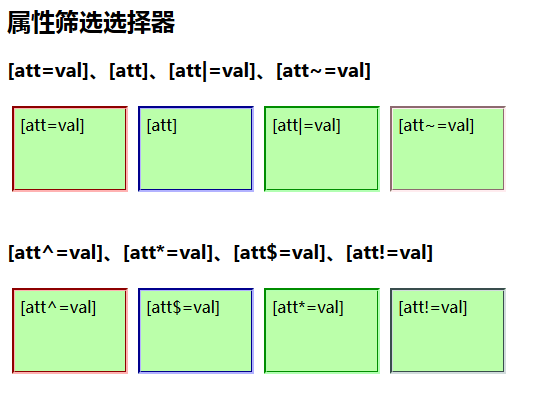# JQuery常用选择器功能与用法实例分析_晚秋_前端开发者

jquery基础回顾

jquery是javascript的一个库，所以二者可以相互转换，例如jquery到Javascript，用\$()获取元素，传给js的var变量。反之js的getElement方法获取var变量后，可以通过\$(var)转化成jquery对象。

JQuery的选择器，

id选择\$(“#id”)，class选择\$(“.class”)，元素选择\$(“elemrnt”)，选择所有\$(“*”)。层级选择器有：子选择器\$(“parent>child”)选中直接子代，后代选择器\$(“ancensterdescendant”)选中所有的后代元素，相邻兄弟选择器\$(“prev+next”)选中prev之后的第一个next节点，一般兄弟选择\$(“prev~siblings”)选中prev之后所有siblings元素。Jquery常用属性选择器如下：

\$(“:first”)

\$(“:last”)

\$(“:eq(n-1)”)

\$(“:gt(n-1)”)

\$(“:lt(n-1)”)

\$(“:even”)

\$(“:odd”)

\$(“:not(selector)”)

\$(“:animated”)

\$(“:visible”)

\$(“:hidden”)

\$(“:first-child”)

\$(“:last-child”)

\$(“:only-child”)

\$(“:nth-child(n)”)

\$(“:nth-last-child(n)”)

First与first-child区别：

```<ul>
<li>第1个ul的第1个li</li>
<li>第1个ul的第2个li</li>
<li>第1个ul的第3个li</li>
</ul>
<ul>
<li>第2个ul的第1个li</li>
<li>第2个ul的第2个li</li>
<li>第2个ul的第3个li</li>
</ul>

```

first表示（所有父元素合并后的）第一个元素；first-child表示（每个父元素的）第一个

`\$('ul li:first')` 返回”第1个ul的第1个li”。 查找所有ul下第一个li元素

`\$("ul li:first-child")` 返回”第1个ul的第1个li”与”第2个ul的第1个li”。 查找每个ul下第一个元素是li元素dom元素。

\$(“:contains(‘text’)”)

\$(“:parent”)

\$(“empty”)

\$(“:has(selector)”)

\$(“[attribute]”)

\$(“[attribute=’value’]”)

\$(“[attribute!=’value’]”)

\$(“[attribute^=’value’]”)

\$(“[attribute\$=’value’]”)

\$(“[attribute*=’value’]”)

\$(“[attribute|=’value’]”)

\$(“[attribute~=’value’]”)

\$(“[attribute1] [attributeN]”)

```<!DOCTYPE html>
<html>
<meta http-equiv="Content-type" content="text/html; charset=utf-8" />
<title>属性筛选选择器</title>
<link rel="stylesheet" href="imooc.css" rel="external nofollow" type="text/css">
<script src="http://libs.baidu.com/jquery/1.9.1/jquery.js"></script>
<body>
<h3>[att=val]、[att]、[att|=val]、[att~=val]</h3>
<div class="left" testattr="true" >
<div class="div" testattr="true" name='p1'>
<a>[att=val]</a>
</div>
<div class="div" testattr="true" p2>
<a>[att]</a>
</div>
<div class="div" testattr="true" name="t-est">
<a>[att|=val]</a>
</div>
<div class="div" testattr="true" name="a b">
<a>[att~=val]</a>
</div>
</div>
<script type="text/javascript">
//查找所有div中，属性name=p1的div元素
\$('div[name=p1]').css("border", "3px groove red");
//查找所有div中，有属性p2的div元素
\$("div[p2]").css("border", "3px groove blue");
//查找所有div中，属性name中包含一个连字符"-"为前缀的div元素
\$('div[name|="t"]').css("border", "3px groove #00FF00");
//查找所有div中，属性name中包含一个连字符"空"和"a"的div元素
\$("div[name~='a']").css("border", "3px groove pink");
</script>
<h3>[att^=val]、[att*=val]、[att\$=val]、[att!=val]</h3>
<div class="left" testattr="true" >
<div class="div" testattr="true" name='start-name'>
<a>[att^=val]</a>
</div>
<div class="div" testattr="true" name='name-end'>
<a>[att\$=val]</a>
</div>
<div class="div" testattr="true" name="attr-test-selector">
<a>[att*=val]</a>
</div>
<div class="div" name="a b">
<a>[att!=val]</a>
</div>
</div>
<script type="text/javascript">
//查找所有div中，属性name的值是用start开头的
\$("div[name^='start']").css("border", "3px groove red");
//查找所有div中，属性name的值是用end结尾的
\$("div[name\$='end']").css("border", "3px groove blue");
//查找所有div中，有属性name中的值包含一个test字符串的div元素
\$("div[name*='test']").css("border", "3px groove #00FF00");
//查找所有div中，有属性testattr中的值没有包含"true"的div
\$("div[testattr!='true']").css("border", "3px groove #668B8B");
</script>
</body>
</html>

```\$(“input:type”)

\$(“:enabled”)

\$(“:disabled”)

\$(“:checked”)

\$(“:selected”)

» 本文链接地址：https://www.rokub.com/71946.html
» 您也可以订阅本站：https://www.rokub.com
64K

• 回顶